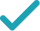Type a math problemSolve for xSteps by Finding Square Root
Steps by Finding Square Root
Take the square root of both sides of the equation.
Solve for ySolution Steps
Swap sides so that all variable terms are on the left hand side.
Solve for x (complex solution)GraphGiving is as easy as 1, 2, 3
Get 1,000 points to donate to a school of your choice when you join Give With Bing
x=\sqrt{y} x=-\sqrt{y}
Take the square root of both sides of the equation.
x^{2}-y=0
Subtract y from both sides.
x=\frac{0±\sqrt{0^{2}-4\left(-y\right)}}{2}
This equation is in standard form: ax^{2}+bx+c=0. Substitute 1 for a, 0 for b, and -y for c in the quadratic formula, \frac{-b±\sqrt{b^{2}-4ac}}{2a}.
x=\frac{0±\sqrt{-4\left(-y\right)}}{2}
Square 0.
x=\frac{0±\sqrt{4y}}{2}
Multiply -4 times -y.
x=\frac{0±2\sqrt{y}}{2}
Take the square root of 4y.
x=\sqrt{y}
Now solve the equation x=\frac{0±2\sqrt{y}}{2} when ± is plus.
x=-\sqrt{y}
Now solve the equation x=\frac{0±2\sqrt{y}}{2} when ± is minus.
x=\sqrt{y} x=-\sqrt{y}
The equation is now solved.
y=x^{2}
Swap sides so that all variable terms are on the left hand side.